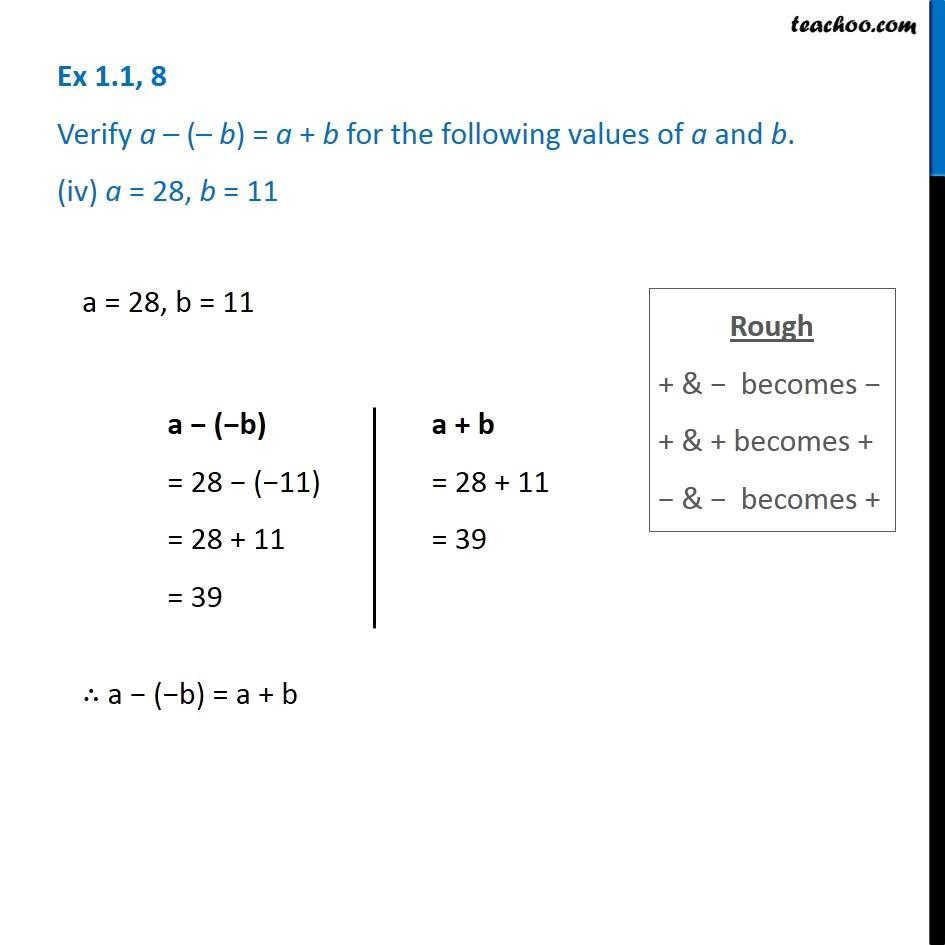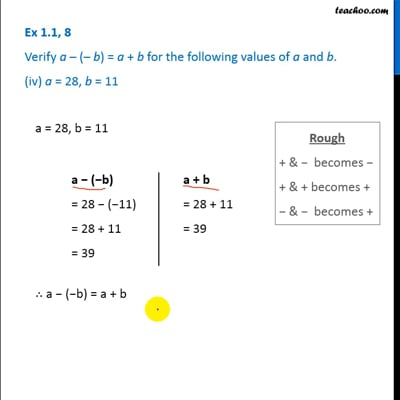Chapter 1 Class 7 Integers
Serial order wiseThis video is only available for Teachoo black users

Learn in your speed, with individual attention - Teachoo Maths 1-on-1 Class

### Transcript

Question 8 Verify a – (– b) = a + b for the following values of a and b. (iv) a = 28, b = 11 a = 28, b = 11 a − (−b) = 28 − (−11) = 28 + 11 = 39 a + b = 28 + 11 = 39 ∴ a − (−b) = a + b